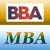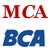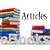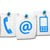Game Theory Introduction

Game theory was developed for the purpose of analyzing competitive situations involving conflicting interests. In other words, game theory is used for decision making under conflicting situations where there are one or more opponents (i.e., players).

For example, chess, poker, etc., are the games which have the characteristics of a competition and are played according to definite rules. Game theory provides solutions to such games, assuming that each of the players wants to maximize his profits and minimize his losses.

How to select the optimal strategy without knowledge of the competitors is the basic problem of playing a game?

The game theory models can be classified into several categories. Some important categories are listed below.

Two-person & N-person games

If the number of players is two, it is known as two-person game. On the other hand, if the number of players is N, it is known as N-person game.

Zero sum & Non-zero sum game

In a zero sum game, the sum of the points won equals the sum of the points lost, i.e., one player wins at the expense of the other. To the contrary, if the sum of gains or losses is not equal to zero, it is either positive or negative, then it is known as non-zero sum game. An example of non-zero sum game is the case of two competing firms each with a choice regarding its advertising campaign. In such a situation, both the firms may gain or loose, though their gain or loss may not be equal.

Games of Perfect and Imperfect information

If the strategy of a player can be discovered by his competitor, then it is known as a perfect information game. In case of imperfect information games no player has complete information and tries to guess the real situation.

Pure & Mixed strategy games

If the players select the same strategy each time, then it is referred to as pure strategy games. If a player decides to choose a course of action for each play in accordance with some particularly probability distribution, it is called mixed strategy game.

Assumptions of Game Theory

• There are finite number of competitors (players).
• The players act reasonably.
• Every player strives to maximize gains and minimize losses.
• Each player has finite number of possible courses of action.
• The choices are assumed to be made simultaneously, so that no player knows his opponent's choice until he has decided his own course of action.
• The pay-off is fixed and predetermined.
• The pay-offs must represent utilities.

Share and Recommend

•MBA & BBA
•BCA & MCA
•Ebooks & Articles
•Contact us